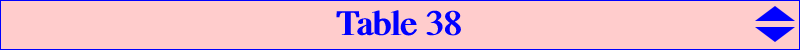This table gives a selection of points whose TRILINEAR coordinates are related to trigonometric functions of the angles A+t, B+t, C+t where t is any real number. The points in cos and sin lie on the Brocard axis. For other properties, see the related cubics K629, K630. Moreover, the circle C(t) with diameter these two points passes through the Brocard points and is obviously centered on the Brocard axis. C(0) is the Brocard circle. C(± π/4) has center X(32) and diameter X(371)X(372). Only one circle is orthogonal to the circumcircle : its center is X(1691) and its diameter is X(1687)X(1688). It is obtained for t = – w/2 ± π/4, see table below. The points in sec and csc lie on the Kiepert hyperbola and they are collinear with X(5). The points in tan and cot lie on the cubic K457. See below for further properties. *** Remark : if the coordinates are supposed to be BARYCENTRIC coordinates, the three loci above are the trilinear polar of X(662), the circum-conic with perspector X(1577), the cubic K267 = cK(#X2, X5 x X99). *** Notations : w is the Brocard angle, P* is the isogonal conjugate of P, [P] is the tangential of P on the cubic K457. See also Table 27. The blue points were added to ETC on June, 05 2008 and the green points on January 10, 2013.cos sin sec csc tan cot notes on the Brocard axis on the Kiepert hyperbola on the cubic K457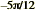X(3364) X(3365) X(3366) X(3367) Q4 Q1 [Q1] = [Q4] = M6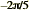X(3368) X(3369) X(3370) X(1140) C2' [C2'] = C1', see note 1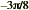X(3371) X(3372) X(3373) X(3374) R4 R1 [R1] = [R4] = M4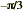X(61) X(16) X(17) X(14) M3 = X(3375) P6 = X(3376) [P6] = M3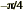X(371) X(372) X(485) X(486) M4 = X(3377) P4 = X(3378) [P4] = [M4] = X(63)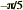X(3379) X(3380) X(3381) X(3382) C1' [C1'] = C2, see note 1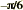X(15) X(62) X(13) X(18) M6 = X(3383) P3 = X(3384) [M6] = P3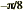X(3385) X(3386) X(3387) X(3388) R2 R3 [R2] = [R3] = P4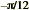X(3389) X(3390) X(3391) X(3392) Q2 Q3 [Q2] = [Q3] = P6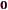X(3) X(6) X(4) X(2) X(19) X(63) [X(63)] = X(19)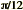X(3365) X(3364) X(3367) X(3366) Q1 Q4 [Q1] = [Q4] = M6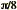X(3372) X(3371) X(3374) X(3373) R1 R4 [R1] = [R4] = M4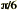X(16) X(61) X(14) X(17) P6 = X(3376) M3 = X(3375) [P6] = M3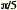X(3393) X(3394) X(5401) X(5402) C1 [C1] = C2', see note 1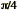X(372) X(371) X(486) X(485) P4 = X(3378) M4 = X(3377) [P4] = [M4] = X(63)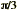X(62) X(15) X(18) X(13) P3 = X(3384) M6 = X(3383) [M6] = P3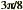X(3386) X(3385) X(3388) X(3387) R3 R2 [R2] = [R3] = P4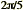X(3395) X(3396) X(3397) X(1139) C2 [C2] = C1, see note 1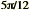X(3390) X(3389) X(3392) X(3391) Q3 Q2 [Q2] = [Q3] = P6 Points related to the Brocard angle w –2w X(3398) X(1691) X(3399) X(1916) M2w = X(3400) M2w* = X(3401) –w X(182) X(32) X(262) X(76) Mw = X(3402) Mw* = X(3403) [Mw] = [Mw*] = P2w –w/2 X(1342) X(1343) X(5403) X(5404) w/2 X(1670) X(1671) X(1677) X(1676) w X(511) X(39) X(98) X(83) Pw = X(3404) Pw* = X(3405) [Pw] = [Pw*] = M2w 2w X(3095) X(3094) X(3406) X(3407) P2w = X(3408) P2w* = X(3409) w + π/6 X(3105) X(3106) w - π/6 X(3104) X(3107) w + π/4 X(3103) X(3102) w - π/4 X(3102) X(3103) w + π/3 X(3107) X(3104) w - π/3 X(3106) X(3105) -w/2 - π/4 X(1687) X(1688) X(2009) X(2010) -w/2 + π/4 X(1688) X(1687) X(2010) X(2009) w/2 - π/4 X(1690) X(1689) w/2 + π/4 X(1689) X(1690) Points related to the angle θ = arctan 2 –θ X(3311) X(1152) X(3316) X(1132) θ X(3312) X(1151) X(3317) X(1131) Points related to the angle U such that cotU = cot(A/2) cot(B/2) cot(C/2) –U X(572) X(58) X(2051) X(10) X(53083) X(21061) U X(573) X(386) X(13478) X(43531) X(53082) X(53081) Points related to the angle V such that tanV = tan(A/2) + tan(B/2) + tan(C/2) = (4R + r) / s –V X(4251) X(13329) X(17758) X(43672) V X(4253) X(991)note 1 : the points C1, C2, C1', C2' = tan(A + k π/5) : : , (k = 1, 2, – 1, – 2) have the remarkable property to coincide with their fourth tangential. There are two other groups of four points with the same property : they have the form tan(A + k π/15) : : , with k = – 2, 1, 4, 7 and k = – 7, – 4, – 2, 1 respectively. note 2 : there are also two groups of three points that coincide with their third tangential. See below. ***Points related to the angle t such that T = cot(t) = k cot(w), w = Brocard angle k cos sin sec csc tan cot on the Brocard axis on the Kiepert hyperbola on the cubic K457 -11 34571 -5 17508 14488 -4 53094 43136 43951 -11/3 14075 -3 5092 5007 14492 10159 -5/2 52519 -2 5085 30435 14484 18840 -5/3 20190 5008 10302 -3/2 12017 -4/3 10541 21309 -1 182 32 262 76 3402 3403 -3/4 22331 43681 -2/3 53093 1384 53099 5485 -3/5 50664 35007 43676 -1/2 5050 3053 14494 2996 -1/3 575 187 7608 671 -1/4 53091 5023 10155 38259 -2/9 15655 32532 -1/5 39561 5206 11669 53105 -4/21 15603 -1/6 53092 5210 53098 41895 -1/7 15516 15513 53108 53106 -1/9 22234 8588 17503 -1/12 5585 -1/15 33698 0 6 3 2 4 63 19 1/9 22330 8589 10185 45103 1/7 15520 15515 11668 53107 1/6 11482 53095 53101 1/5 5097 37512 53104 53109 5/21 15602 1/4 5093 15815 53103 18845 2/7 18844 1/3 576 574 7607 598 2/5 5102 18843 3/7 31652 1/2 1351 5013 7612 5395 3/5 37517 53096 53102 2/3 11477 5024 43537 18842 3/4 44456 22332 1 511 39 98 83 3404 3405 4/3 53097 47586 3/2 33878 5/3 52987 53100 2 1350 9605 3424 18841 8/3 22246 3 3098 7772 14458 43527 4 31884 5 14810 5041 9 41940 column 1 column 2 column 3 column 4 column 5 column 6Remark 1 : recall that the points in a same line and columns {1,3}, {2,4}, {5,6} are pairs of isogonal conjugates. Remark 2 : in columns 1 or 2, two opposite values of k correspond to two points inverse in the Brocard circle and the same value of k in both columns corresponds to two points inverse in an imaginary circle with center X(39). These two latter points are Psi_X39 conjugates, see details here. Two opposite values of k, one in column 1 and the other in column 2, correspond to two points inverse in the circle with diameter X(371), X(372). More generally, two values of k, one k1 in column 1 and the other k2 in column 2, whose quotient is k1/k2 = –K, correspond to two points inverse in a circle orthogonal to the Brocard circle. All these circles are in a pencil with radical axis the perpendicular bisector of X(3)X(6). For instance, • with K = 3, we obtain the Schoute circle with diameter X(15), X(16). • with K = 1/3, we obtain the circle with diameter X(61), X(62). • with K = 1, we obtain the circle with diameter X(371), X(372). • with K = 4, we obtain the circle with diameter X(1151), X(1152). • with K = –1, we obtain the imaginary circle above with diameter X(39641), X(39642), the imaginay foci of the Brocard inellipse. Remark 3 : in columns 3 or 4, two opposite values of k correspond to two points aligned with X(6) and the same value of k in both columns corresponds to two points aligned with X(5). Two opposite values of k, one in column 3 and the other in column 4, correspond to two points aligned with X(3). Remark 4 : for every value of k, the lines passing through two points in columns 1, 3 and two points in columns 2, 4 (which are pairs of isogonal conjugates) envelope the quartic Q073. These two lines meet at Z1 on the Kiepert hyperbola. Remark 5 : for every value of k, the lines passing through two points in columns 1, 4 and two points in columns 2, 3 envelope the quartic Q183. These two lines also meet at Z2 on the Kiepert hyperbola. These two points Z1, Z2 and X(5) are collinear. Remark 6 : The line passing through two points in columns 5, 6 (which are isogonal conjugates) envelopes the pivotal conic of K457.Properties of points on the cubic K457 With T = tan(t), we have tan(A+t) = (tanA + T)/(1 – T tanA) which is the first trilinear coordinate of a point S(T) on K457. It is easy to see that : (1). S(T), S(–T) and X(19) are collinear. S(T) and S(–T) are said to be opposite points on the cubic. (2). S(T), S(1/T) and X(63) are collinear. S(T) and S(1/T) are said to be inverse points on the cubic. (3). S(–1/T) is the isogonal conjugate of S(T), thus the isogonal conjugate of a point on the cubic is the opposite of its inverse and vice versa. Note that these four points are not always distinct. *** More generally, we consider two (distinct or not) points S1 = S(T1) and S2 = S(T2) with trilinear coordinates of the form tan(A+t1) : : and tan(A+t2) : : respectively. The line S1S2 meets the cubic K457 again at S3 = S(T3) = tan(A+t3) : : such that T3 = (T1+T2)/(T1T2–1) or, equivalently, t1+t2+t3 = 0 (mod. π). In particular, – when S1 = S2 = S(T) = tan(A+t) : : , this point S3 is the isogonal conjugate of the tangential [S] of S and then [S] = S(2T/(T^2-1)) or tan(A–2t): : . It easily follows that the isogonal conjugate points S(T) and S(–1/T) share the same tangential. – when t1 = t2 = t3 = 0 (mod. π/3), we obtain the three real inflexion points of the cubic (there are no other inflexion points since the cubic is unicursal). – when t1 = – t2, we must have t3 = 0 : this is property (1). – when t2 = π/2 – t1, we must have t3 = π/2 : this is property (2). This gives an easy way to showing collinearities of points on the cubic :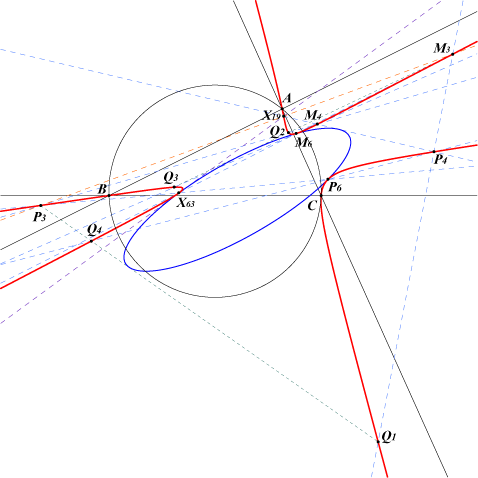• Q1 = tan(A+π/12) : : , since π/12 = –(π/4–π/3) = –(π/6–π4) we have Q1 = P4M3 /\ P6M4 and similarly • Q2 = tan(A–π/12) : : = X(19)Q1 /\ P4M6 /\ P3M4, • Q3 = tan(A+5π/12) : : = tan(A–7π/12) : : = Q2* = X(63)Q1 /\ M3Q2 /\ M4M6 /\ P4P3 /\ X(19)Q4, • Q4 = tan(A–5π/12) : : = tan(A+7π/12) : : = Q1* = X(63)Q2 /\ P3Q1 /\ M4M3 /\ P4P6 /\ X(19)Q3. The common tangential of Q1 and Q4 is obviously M6 and that of Q2 and Q3 is P6.Properties related to tangentials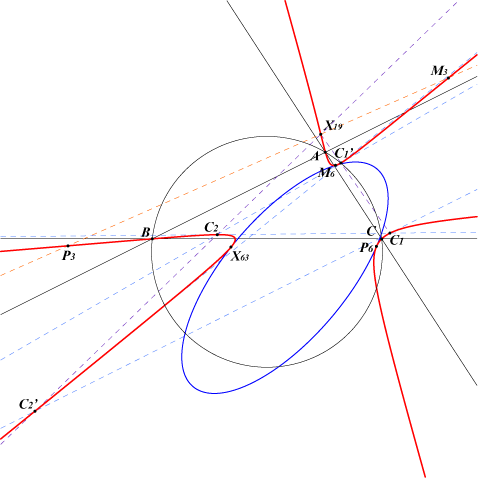• C1 = tan(A+π/5) : : • C2 = tan(A+2π/5) : : • C1' = tan(A–π/5) : : • C2' = tan(A–2π/5) : : Each of these four points is the tangential of another of these same points. Their order is represented by the following diagram. [C2] = C1, etc. Recall that each of these four points coincide with its fourth tangential.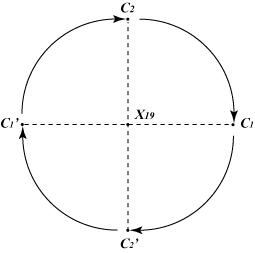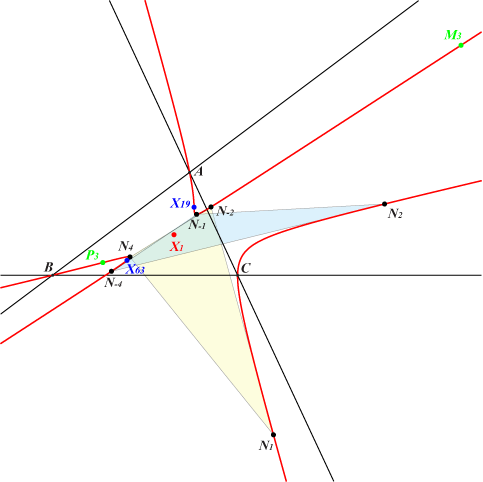Let Nk be the point with first trilinear tan(A + k π/9) with k in {-4, -3, ..., 3, 4}. There are 9 such points among them the three inflexion points N0 = X(19), N3 = P3 = X(3384), N-3 = M3 = X(3375). The remaining 6 points form two groups of 3 points such that the tangential of one point in a group is another point of the same group. These groups are : • N1 - N-2 - N4 • N-1 - N2 - N-4 Recall that : • Nk and N-k are collinear with X(19), • Nk and N3-k are collinear with X(3375), • Nk and N-3-k are collinear with X(3384). The third tangential of each of these 6 points Nk is Nk itself.The related points 1 + cos(A+t) : 1 + cos(B+t) : 1 + cos(C+t) Let us denote by X(t) the point with trilinear coordinates 1 + cos(A+t) : 1 + cos(B+t) : 1 + cos(C+t). In general, X(t) can be associated with seven other points with similar coordinates unless t is 0 or π/4 (mod. π/2) in which case there are only three of them. The table below shows these points and a selection of some special usual cases. When the point is not mentioned in ETC, a SEARCH number is given.point and its first coordinate t = 0 t = π/4 t = w t = π/6 t = 2w X(t) 1 + cos(A+t) X(55) X(π/4) : 3.4111901068237 X(3237) X(π/6) : 3.1500183826781 X(12836) X(t ± π) 1 - cos(A+t) X(56) X(-3π/4) : 0.81516326357652 X(3238) X(-5π/6) : 0.60461376364682 X(12837) X(t - π/2) 1 + sin(A+t) X(1124) X(-π/4) : 1.9821946162931 X(2007) X(-π/3) = X(7005) X(12841) X(t + π/2) 1 - sin(A+t) X(1335) X(3π/4) : 0.3232374569376 X(2008) X(2π/3) = X(203) X(12840) X(-t) 1 + cos(A-t) X(55) X(-π/4) : 1.9821946162931 X(1672) X(-π/6) : 2.1864880808192 X(10799) X(-t ± π) 1 - cos(A-t) X(56) X(3π/4) : 0.3232374569376 X(1673) X(5π/6) : 0.021151202676385 X(12835) X(-t + π/2) 1 - sin(A-t) X(1335) X(π/4) : 3.4111901068237 X(3236) X(π/3) = X(7006) X(12838) X(-t - π/2) 1 + sin(A-t) X(1124) X(-3π/4) : 0.81516326357652 X(3235) X(-2π/3) = X(202) X(12839)Properties of these points • For any real number t, the point X(t) lies on the inscribed ellipse (C) whose perspector is X(59), the isogonal conjugate of the Feuerbach point X(11). Its center is X(13006). Apart the points already mentioned in the table above, (C) also contains the points X(181), X(215), X(1362), X(1397), X(1682), X(6056), X(7066). (C) is a diagonal conic with respect to the triangle IOK = X(1)X(3)X(6) and more generally with respect to any triangle with vertices X(1), Y(T), Z(T) where Y(T), Z(T) are two points on the Brocard axis with trilinears sin(A+T) : : and cos(A+T) : : . These points are conjugated in the Kiepert hyperbola, in (C) and therefore in any conic of the pencil generated by these two latter conics. See K629 for further details. • If t1 and t2 are distinct (mod. 2π) then the line X(t1), X(t2) meets the Brocard axis at Y((t1+t2)/2) = sin(A + (t1+t2)/2) : : . In other words, for any real numbers t and T, the points X(t), X(-t + 2T) and Y(T) are collinear. • The tangents at X(t), X(-t + 2T) to (C) meet at a point lying on the line I, Z(T). • If we take four real numbers t1, t2, t3, t4 such that t1 + t2 = t3 + t4 = 2T (mod. 2π) then the lines X(t1)X(t2) and X(t3)X(t4) meet on the Brocard axis at the point Y(T). The table at the top of the page gives Y(T) according to the value of T. For example, we have the following collinearities : X(3)-X(t)-X(-t ± π); X(6)-X(t)-X(-t); X(371)-X(t)-X(-t +π/2); X(372)-X(t)-X(-t - π/2); etc. • X(t), X(t ± π) are collinear with the incenter I. See below. • More generally, for a given T ≠ 0 (mod. π), the line passing through X(t) and X(t + T) envelopes a conic (CT) with center on the line X(1), X(39) which belongs to a pencil generated by the Brocard axis (counted twice) and (C). When T = ±π, (CT) splits into two imaginary lines secant at X(1). See the generalization below.Connection with the Tucker circles(with the collaboration of Peter Moses) As already said, the line X(t), X(t + π) passes through the incenter I and meets the Brocard axis at Y. X(t), X(t + π) are the centers of similitude of the incircle (I) and the Tucker circle with center Y. Any Y on the Brocard axis has its 1st trilinear coordinate of the form cos(A – 𝝓) where 𝝓 is a real number called the parameter of the Tucker circle (Gallatly). Two points Y, Y' with parameters 𝝓, 𝝓' such that 𝝓 + 𝝓' = 0 (mod. π) are inverses in the Brocard circle hence harmonically conjugates with respect to X(3), X(6). The insimilicenter Si is then 1 + cos(A – 𝝓) : : and the exsimilicenter Se is 1 – cos(A – 𝝓) : : (trilinear coordinates).For example, with 𝝓 = 0, we obtain : Y = cosA : : = X(3), Si = 1 + cosA : : = X(55), Se = 1 – cosA : : = X(56). *** There are four points X(t) lying on (I) and obviously on (C), see figure above. One of them is X(1362) corresponding to the Tucker circle (Co) with center X(1)X(1362)/\ X(3)X(6). The three remaining points are A', B', C' lying on the cevian lines of X(3271). The three corresponding Tucker circles (Ca), (Cb), (Cc) have their centers at the extraversions of X(970) which are the intersections of the Brocard axis and the lines passing through X(1) and A', B', C' respectively. Each of these three Tucker circles is tangent internally to the incircle and externally to the two corresponding excircles. For instance (Ca) is tangent to (I) at A' and to the excircles (Ib), (Ic) at Ab, Ac respectively. Recall that X(970) is the center of the Apollonius circle (which is also a Tucker circle) tangent to the three excircles and encompassing them. The corresponding points on (C) are X(181), X(1682). Further properties of the triangle A'B'C' • A'B'C' is perspective at X(1362) to the cevian triangle of X(651) and to the anticevian triangle of X(665). • A‘B’C’ is perspective to the intangents triangle at X(3022), the incircle antipode of X(1362). • A'B'C' is perspective to the anticevian triangles of X(651), X(1362) and to the circumcevian triangle of X(513) with unlisted perspectors. • A'B'C' is directly similar to the cevian triangle of X(190). Other Tucker circles with center on the Brocard axis and centers of similitude on (C) • circumcircle : X(3) – X(55), X(56). • second Lemoine circle : X(6) – X(1124), X(1335). • Gallatly circle : X(39) – X(2007), X(2008). • first Lemoine circle : X(182) – X(1672), X(1673). • unknown (?) circle : X(32) – X(3235), X(3236). The other Tucker circles (Kenmotu, Taylor, third Lemoine) do not correspond to ETC listed centers of similitude.Generalization : the points k + cos(A+t) : k + cos(B+t) : k + cos(C+t) Let us denote by Xk(t) the point with trilinear coordinates k + cos(A+t) : k + cos(B+t) : k + cos(C+t). For a given value of k, Xk(t) lies on a conic (Ck) which belongs to a pencil of conics generated by the Brocard axis counted twice (obtained with k = 0) and the inscribed conic (C) above (obtained with k = 1). This pencil also contains a conic which is the union of two imaginary lines secant at X(1) and passing through the imaginary intersections of the Brocard axis with the Kiepert hyperbola. These points are isogonal conjugates with midpoint X(39) hence they are the imaginary foci of the Brocard ellipse. Note that two opposite values of k correspond to the same conic (Ck) hence it is sufficient to take k > 0 in the sequel. When k varies, the locus of the center of (Ck) is the line passing through X1, X39, X291, X292, X1009, X1015, X1018, X1500, X2007, X2008, X2108, X2275, X2276, X2295, X3097, X3208, etc. Apart the two decomposed and the inscribed conics above, the pencil contains one bicevian conic (which is a hyperbola), one parabola, one rectangular hyperbola, all not very interesting.Points k + tan(A+t) : k + tan(B+t) : k + tan(C+t) See the page K457.